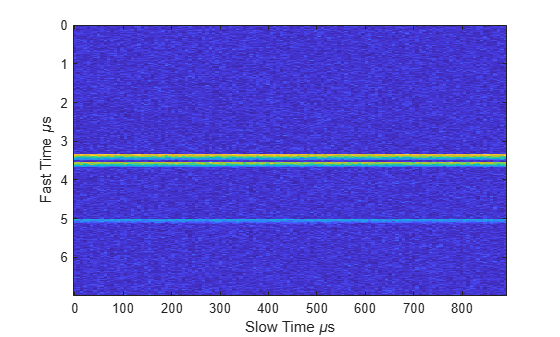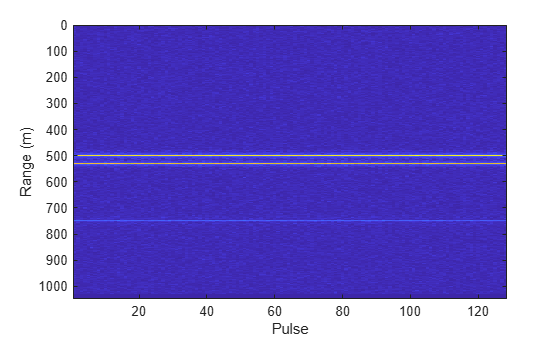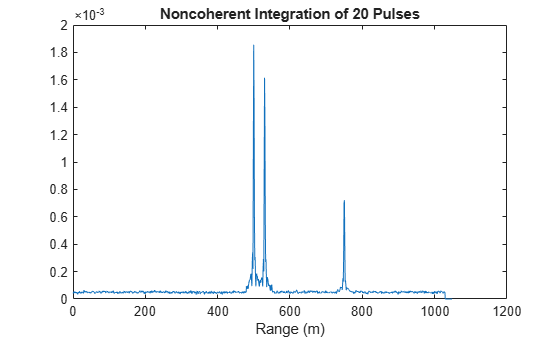# step

System object: phased.RangeResponse
Package: phased

Range response

## Syntax

[resp,rnggrid] = step(response,x)
[resp,rnggrid] = step(response,x,xref)
[resp,rnggrid] = step(response,x,coeff)

## Description

Note

Instead of using the step method to perform the operation defined by the System object™, you can call the object with arguments, as if it were a function. For example, y = step(obj,x) and y = obj(x) perform equivalent operations.

[resp,rnggrid] = step(response,x) computes the range response, resp, for the input signal, x, and the range values, rnggrid, corresponding to the response. This syntax applies when you set RangeMethod to 'FFT' and DechirpInput to false. This syntax assumes that the input signal has already been dechirped. This syntax is most commonly used with FMCW signals.
[resp,rnggrid] = step(response,x,xref) computes the range response of the input signal, x using the reference signal, xref. This syntax applies when you set RangeMethod to 'FFT' and DechirpInput to true. Often, the reference signal is the transmitted signal. This syntax assumes that the input signal has not been dechirped. This syntax is most commonly used with FMCW signals.

example

[resp,rnggrid] = step(response,x,coeff) computes the range response of x using the matched filter coeff. This syntax applies when you set RangeMethod to 'Matched filter'. This syntax is most commonly used with pulsed signals.

Note

The object performs an initialization the first time the object is executed. This initialization locks nontunable properties and input specifications, such as dimensions, complexity, and data type of the input data. If you change a nontunable property or an input specification, the System object issues an error. To change nontunable properties or inputs, you must first call the release method to unlock the object.

## Input Arguments

expand all

Range response, specified as a phased.RangeResponse System object.

Example: phased.RangeResponse

Input radar data cube, specified as a complex-valued K-by-1 column vector, a K-by-L matrix, or K-by-N-by-L array.

• K is the number of fast-time or range samples.

• N is the number of independent spatial channels such as sensors or directions.

• L is the slow-time dimension that corresponds to the number of pulses or sweeps in the input signal.

See Radar Data Cube.

Each K-element fast-time dimension is processed independently.

For FMCW waveforms with a triangle sweep, the sweeps alternate between positive and negative slopes. However, phased.RangeResponse is designed to process consecutive sweeps of the same slope. To apply phased.RangeResponse for a triangle-sweep system, use one of the following approaches:

• Specify a positive SweepSlope property value, with x corresponding to upsweeps only. After obtaining the Doppler or speed values, divide them by 2.

• Specify a negative SweepSlope property value, with x corresponding to downsweeps only. After obtaining the Doppler or speed values, divide them by 2.

The size of the first dimension of the input matrix can vary to simulate a changing signal length. A size change can occur, for example, in the case of a pulse waveform with variable pulse repetition frequency.

Data Types: single | double
Complex Number Support: Yes

Reference signal used for dechirping, specified as a complex-valued K-by-1 column vector. The number of rows must equal the length of the fast-time dimension of x.

#### Dependencies

To enable this input argument, set the value of RangeMethod to 'FFT' and DechirpInput to true.

Data Types: single | double
Complex Number Support: Yes

Matched filter coefficients, specified as a complex-valued P-by-1 column vector. P must be less than or equal to K. K is the number of fast-time or range sample.

#### Dependencies

To enable this input argument, set the value of RangeMethod to 'Matched filter'.

Data Types: double
Complex Number Support: Yes

## Output Arguments

expand all

Range response data cube, returned as one of the following:

• Complex-valued M-element column vector

• Complex-valued M-by-L matrix

• Complex-valued M-by-N by-L array

The value of M depends on the type of processing

RangeMethod PropertyDechirpInput PropertyValue of M
'FFT'false

If you set the RangeFFTLength property to 'Auto', M = K, the length of the fast-time dimension of x. Otherwise, M equals the value of the RangeFFTLength property.

trueM equals the quotient of the number of rows, K, of the input signal by the value of the decimation factor, D, specified in DecimationFactor.
'Matched filter'n/aM = K, the length of the fast-time dimension of x.

Data Types: single | double
Complex Number Support: Yes

Range values along range dimension, returned as a real-valued M-by-1 column vector. rnggrid defines the ranges corresponding to the fast-time dimension of the resp output data cube. M is the length of the fast-time dimension of resp. Range values are monotonically increasing and equally spaced. Units are in meters.

Example: [0,0.1,0.2,0.3]

Data Types: single | double

## Examples

expand all

Compute the radar range response of three targets by using the phased.RangeResponse System object™. The transmitter and receiver are collocated isotropic antenna elements forming a monostatic radar system. The transmitted signal is a linear FM waveform with a pulse repetition interval of 7.0 μs and a duty cycle of 2%. The operating frequency is 77 GHz and the sample rate is 150 MHz.

fs = 150e6; c = physconst('LightSpeed'); fc = 77e9; pri = 7e-6; prf = 1/pri;

Set up the scenario parameters. The radar transmitter and receiver are stationary and located at the origin. The targets are 500, 530, and 750 meters from the radars on the x-axis. The targets move along the x-axis at speeds of −60, 20, and 40 m/s. All three targets have a nonfluctuating radar cross-section (RCS) of 10 dB.

Create the target and radar platforms.

Numtgts = 3; tgtpos = zeros(Numtgts); tgtpos(1,:) = [500 530 750]; tgtvel = zeros(3,Numtgts); tgtvel(1,:) = [-60 20 40]; tgtrcs = db2pow(10)*[1 1 1]; tgtmotion = phased.Platform(tgtpos,tgtvel); target = phased.RadarTarget('PropagationSpeed',c,'OperatingFrequency',fc, ... 'MeanRCS',tgtrcs); radarpos = [0;0;0]; radarvel = [0;0;0]; radarmotion = phased.Platform(radarpos,radarvel);

Create the transmitter and receiver antennas.

txantenna = phased.IsotropicAntennaElement; rxantenna = clone(txantenna);

Set up the transmitter-end signal processing. Create an upsweep linear FM signal with a bandwidth of half the sample rate. Find the length of the pri in samples and then estimate the rms bandwidth and range resolution.

bw = fs/2; waveform = phased.LinearFMWaveform('SampleRate',fs, ... 'PRF',prf,'OutputFormat','Pulses','NumPulses',1,'SweepBandwidth',fs/2, ... 'DurationSpecification','Duty cycle','DutyCycle',0.02); sig = waveform(); Nr = length(sig); bwrms = bandwidth(waveform)/sqrt(12); rngrms = c/bwrms;

Set up the transmitter and radiator System object properties. The peak output power is 10 W and the transmitter gain is 36 dB.

peakpower = 10; txgain = 36.0; transmitter = phased.Transmitter( ... 'PeakPower',peakpower, ... 'Gain',txgain, ... 'InUseOutputPort',true); radiator = phased.Radiator( ... 'Sensor',txantenna, ... 'PropagationSpeed',c, ... 'OperatingFrequency',fc);

Create a free-space propagation channel in two-way propagation mode.

channel = phased.FreeSpace( ... 'SampleRate',fs, ... 'PropagationSpeed',c, ... 'OperatingFrequency',fc, ... 'TwoWayPropagation',true);

Set up the receiver-end processing. The receiver gain is 42 dB and noise figure is 10.

collector = phased.Collector( ... 'Sensor',rxantenna, ... 'PropagationSpeed',c, ... 'OperatingFrequency',fc); rxgain = 42.0; noisefig = 10; receiver = phased.ReceiverPreamp( ... 'SampleRate',fs, ... 'Gain',rxgain, ... 'NoiseFigure',noisefig);

Loop over 128 pulses to build a data cube. For each step of the loop, move the target and propagate the signal. Then put the received signal into the data cube. The data cube contains the received signal per pulse. Ordinarily, a data cube has three dimensions, where last dimension corresponds to antennas or beams. Because only one sensor is used in this example, the cube has only two dimensions.

The processing steps are:

1. Move the radar and targets.

2. Transmit a waveform.

3. Propagate the waveform signal to the target.

4. Reflect the signal from the target.

5. Propagate the waveform back to the radar. Two-way propagation mode enables you to combine the returned propagation with the outbound propagation.

6. Receive the signal at the radar.

7. Load the signal into the data cube.

Np = 128; cube = zeros(Nr,Np); for n = 1:Np [sensorpos,sensorvel] = radarmotion(pri); [tgtpos,tgtvel] = tgtmotion(pri); [tgtrng,tgtang] = rangeangle(tgtpos,sensorpos); sig = waveform(); [txsig,txstatus] = transmitter(sig); txsig = radiator(txsig,tgtang); txsig = channel(txsig,sensorpos,tgtpos,sensorvel,tgtvel); tgtsig = target(txsig); rxcol = collector(tgtsig,tgtang); rxsig = receiver(rxcol); cube(:,n) = rxsig; end

Display the image of the data cube containing signals per pulse.

imagesc([0:(Np-1)]*pri*1e6,[0:(Nr-1)]/fs*1e6,abs(cube)) xlabel('Slow Time {\mu}s') ylabel('Fast Time {\mu}s')Create a phased.RangeResponse System object in matched filter mode. Then, display the range response image for the 128 pulses. The image shows range vertically and pulse number horizontally.

matchingcoeff = getMatchedFilter(waveform); ndop = 128; rangeresp = phased.RangeResponse('SampleRate',fs,'PropagationSpeed',c); [resp,rnggrid] = rangeresp(cube,matchingcoeff); imagesc([1:Np],rnggrid,abs(resp)) xlabel('Pulse') ylabel('Range (m)')Integrate 20 pulses noncoherently.

intpulse = pulsint(resp(:,1:20),'noncoherent'); plot(rnggrid,abs(intpulse)) xlabel('Range (m)') title('Noncoherent Integration of 20 Pulses')## Version History

Introduced in R2017a